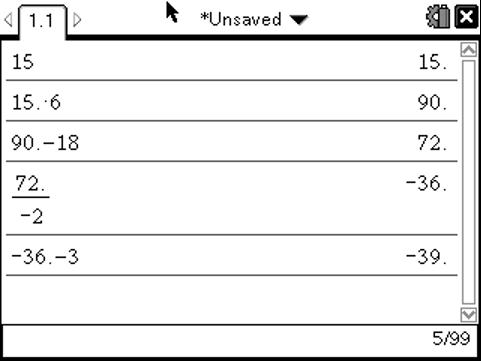# Activities

•• ##### Subject Area

• Math: PreAlgebra: Equations
• Math: PreAlgebra: Number Sense and Operations
• Math: Algebra I: Equations

• ##### Author6-8
9-12

30 Minutes

• ##### Device
• TI-Nspire™
•TI-Nspire™ CX/CX II

3.2

## Number Trick Equations#### Activity Overview

This activity is basically using undo charts to solve complex equations.

This is a great way for teachers to use Nspire on it's most basic level. Have the students go to a calculator scratchpad page by pressing Home>A. The students use the undo charts (Jerry Murdock, Discovering Algebra) to find the value of x. The equations that the students are solving are under the 'guise' of being number tricks. Students are to then record their calculations on the screens of their handhelds.